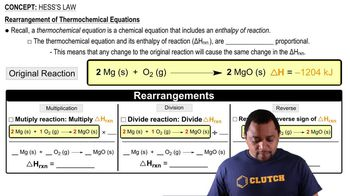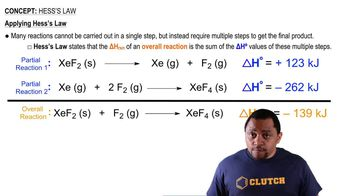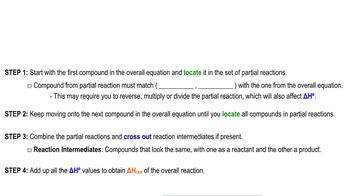Start typing, then use the up and down arrows to select an option from the list.
2:30 minutes
Problem 97
Textbook Question

# Set up a Hess's law cycle, and use the following information to calculate ΔH°f for aqueous nitiric acid, HNO3(aq). You will need to use fractional coefficients for some equations. 3 NO2(g) + H2O(l) → 2 HNO3(aq) + NO(g) ΔH° = -137.3 kJ 2 NO(g) + O2(g) → 2 NO2(g) ΔH° = -116.2 kJ 4 NH3(g) + 5 O2(g) → 4 NO (g) + 6 H2O(l) ΔH° = -1165.2 kJ NH3(g) ΔH°f = -46.1 kJ/mol H2O(l) ΔH°f = -285.8 kJ/molVerified Solution
This video solution was recommended by our tutors as helpful for the problem above.
160views

### Watch next

Master Hess's Law with a bite sized video explanation from Jules Bruno

Start learning03:1101:3902:0305:4307:46